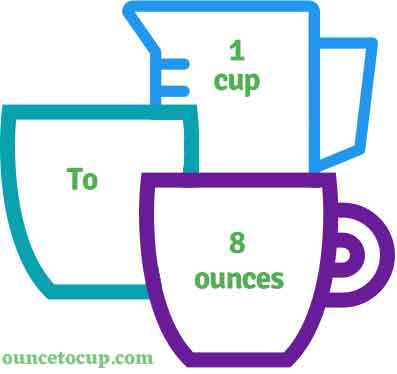# Ounces in a Cup (cup to oz conversion)

Are you cooking your favorite dish? The detailed chart in the recipe includes the calculation of the 1 cup to ounce conversion.  Don't worry; use this calculator to determine how many 1 cup equals ounces in a minute.  This 1 cup to oz converter gives an exact measurement for any recipe you prepare.

Cup Value:

cup

Ounce Value:

oz

1 Cup = 8 Ounce
(1 cup = 8 oz)

Try our auto 1 Cup to Ounce Calculator (Without Convert Button), Just change the first field value and you got final value.## How many oz is a cup?

We know that the volume value of 1 cup is equal to 8 oz. If you want to convert 1 cup to an equal number of oz, just multiply the volume value by 8 or divide the value by 29.5735. Hence, 1 cup is equal to 8 oz.

The Answer is: 1 US Cups = 8 US Fluid Ounce

1 cup = 8 oz

Many of them try to search or find an answer for what is 1 cups in oz? So, we’ll start with 1 cup to oz conversion to know how big is 1 cup.

## How To Calculate 1 cup to oz?

To calculate 1 cups to an equal number of fluid ounce, simply follow the steps below.

Cups to Fluid Ounces formula is:

Fluid Ounce = Cup * 8

Assume that we are finding out how many oz were found in 1 fl cup of water, multiply by 8 to get the result.

Applying to Formula: oz = 1 cup * 8 = 8 oz.

## How To Convert cup to oz?

• To convert cups to oz,
• Simply multiply the cup value by 8 (or) simply divide them by 29.5735.
• Applying to the formula, oz = 1 cups * 8 [1x8].
• Hence, 1 cups is equal to 8 oz.

## Some quick table references for cup to ounce conversions:

Cup [c]Ounce [oz]
1 cup8 oz
2 cup16 oz
3 cup24 oz
4 cup32 oz
5 cup40 oz
6 cup48 oz
7 cup56 oz
8 cup64 oz
9 cup72 oz
10 cup80 oz
11 cup88 oz
12 cup96 oz
13 cup104 oz
14 cup112 oz
15 cup120 oz

## Reverse Calculation: How many cups are in a oz?

• To convert 1 oz to cup,
• Simply divide the 1 oz by 8.
• Then, applying the formula, cup = 1 oz / 8 [1/8 = 0.125].
• Hence, 1 ounce is equal to 0.125 cup.